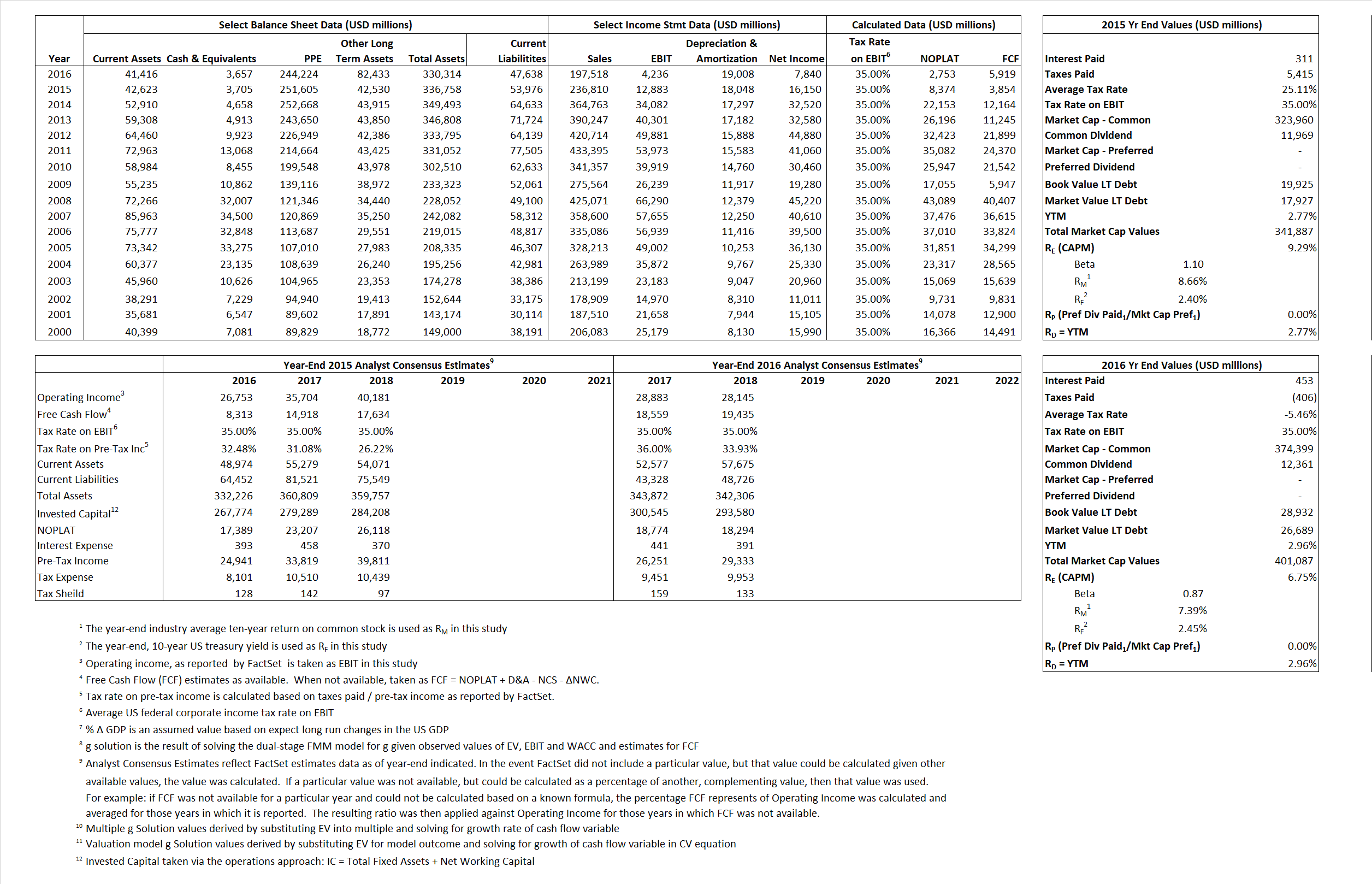# Exxon Mobil Corporation

## Analyst Listing

The following analysts provide coverage for the subject firm as of May 2016:

 Broker Analyst Analyst Email Tudor Pickering Holt & Co. Anish Kapadia akapadia@tudorpickering.com Scotia Howard Weil Blake Fernandez blake.fernandez@scotiabank.com Evercore ISI Douglas Terreson doug.terreson@evercoreisi.com Credit Suisse Edward Westlake edward.westlake@credit-suisse.com Piper Jaffray Guy Baber guy.a.baber@simmonspjc.com Wolfe Research Paul Sankey psankey@wolferesearch.com Raymond James Pavel Molchanov pavel.molchanov@raymondjames.com Wells Fargo Securities Roger D. Read roger.read@wellsfargo.com Cowen & Company Sam Margolin sam.margolin@cowen.com

## Primary Input Data## Derived Input Data

### Equational Form

Net Operating Profit Less Adjusted Taxes NOPLAT 8,374 2,759$NOPLAT\, =\, EBIT\, x\, (1 \,-\, Avg \,\,Tax\,\, Rate\,\, on\,\, EBIT)$
Free Cash Flow FCF 3,854 5,919$FCF\,=NOPLAT\,+\,Non-Cash\,Expenses-\Delta NWC\,-\,NCS$
Tax Shield TS 78 (25)$TS\,=\,Interest\,\,Paid\,\,x\,\, Avg \,\,Tax\,\,Rate\,\, on\,\, Pre-Tax\,\, Income$
Invested Capital IC 282,782 320,435$IC\,=\,Fixed\,\,Operating\,\,Assets\,\,+\,\,Net\,\, Working\,\, Capital$
Return on Invested Capital ROIC 2.96% 0.86%$ROIC\,=\,\frac { NOPLAT }{ IC }$
Net Investment NetInv 15,970 56,661$NetInv\,=\,{ {IC}_{1}}-{{IC}_{0}}+Depreciation$
Investment Rate IR 190.71% 2,057.86%$IR\,=\,\frac {NetInv}{NOPLAT}$
Weighted Average Cost of Capital
WACCMarket 8.91% 6.51%$WACC\,=\,\frac { E }{ V } { R }_{ E }\,+\,\frac { P }{ V } { R }_{ P }\,+\,\frac { D }{ V } { R }_{ D }\left( 1- Avg\,\, Tax\,\,Rate\,\,on\,\,Pre-Tax\,\,Income \right)$
WACCBook    9.19%    7.50%
Enterprise value
EVMarket 338,182  397,430$EV\,=\,Market\,\,Cap\,\,Equity\,+\,\,Long\,\,Term\,\,Debt\,-\,Cash$
EVBook  340,228  399,674
Long-Run Growth
g = IR x ROIC
5.65%    17.68% Long-run growth rates of the income variable are used in the Continuing Value portion of the valuation models.
g = %$\Delta$ GDP    2.50%     2.50%
Margin from Operations M   5.44%    2.14%$M\,\,=\,\,\frac{EBIT}{SALES}$
Depreciation/Amortization Rate D  58.35%    81.78%$D\,\,=\,\,\frac{D+A}{EBITDA}$

## Valuation Multiple Outcomes

The outcomes presented in this study are the result of original input data, derived data, and synthesized inputs.

### model g solution

12/31/2015 12/31/2016 12/31/2015 12/31/2016 12/31/2015 12/31/2016

EV/SALES$\frac {EV}{Sales} \,= \,\frac{ROIC\, -\, g}{ROIC\,(WACC\,-\,g)}\,(1\,-\,T)\,(M)$

1.43  2.01  39.26%  30.01%  24.15%  20.38%

EV/EBITDA$\frac {EV}{EBITDA} \,= \,\frac{ROIC\, -\, g}{ROIC\,(WACC\,-\,g)}\,(1\,-\,T)\,(1\,-\,D)$

10.93 17.10 39.26% 30.01% 24.15% 20.38%

EV/NOPLAT$\frac {EV}{NOPLAT} \,= \,\frac{ROIC\, -\, g}{ROIC\,(WACC\,-\,g)}$

40.38 144.34 39.26% 30.01% 24.15% 20.38%

EV/FCFOPS$\frac {EV}{FCF_{OPS}} \,= \,\frac{ROIC\, -\, g}{ROIC\,(WACC\,-\,g)}\,(1\,-\,T)$

87.75 67.14 39.26% 30.01% 24.15% 20.38%

EV/EBIT$\frac {EV}{EBIT} \,= \,\frac{ROIC\, -\, g}{ROIC\,(WACC\,-\,g)}\,(1\,-\,T)$

26.25 93.82 39.26% 30.01% 24.15% 20.38%

EV/IC$\frac {EV}{IC} \,= \,\frac{ROIC\, -\, g}{WACC\,-\,g}$

1.20 1.24 39.26% 30.01% 24.15% 20.38%